# Pandas Groupby Aggregates with Multiple Columns

Pandas groupby is a powerful function that groups distinct sets within selected columns and aggregates metrics from other columns accordingly.

Performing these operations results in a pivot table, something that’s very useful in data analysis.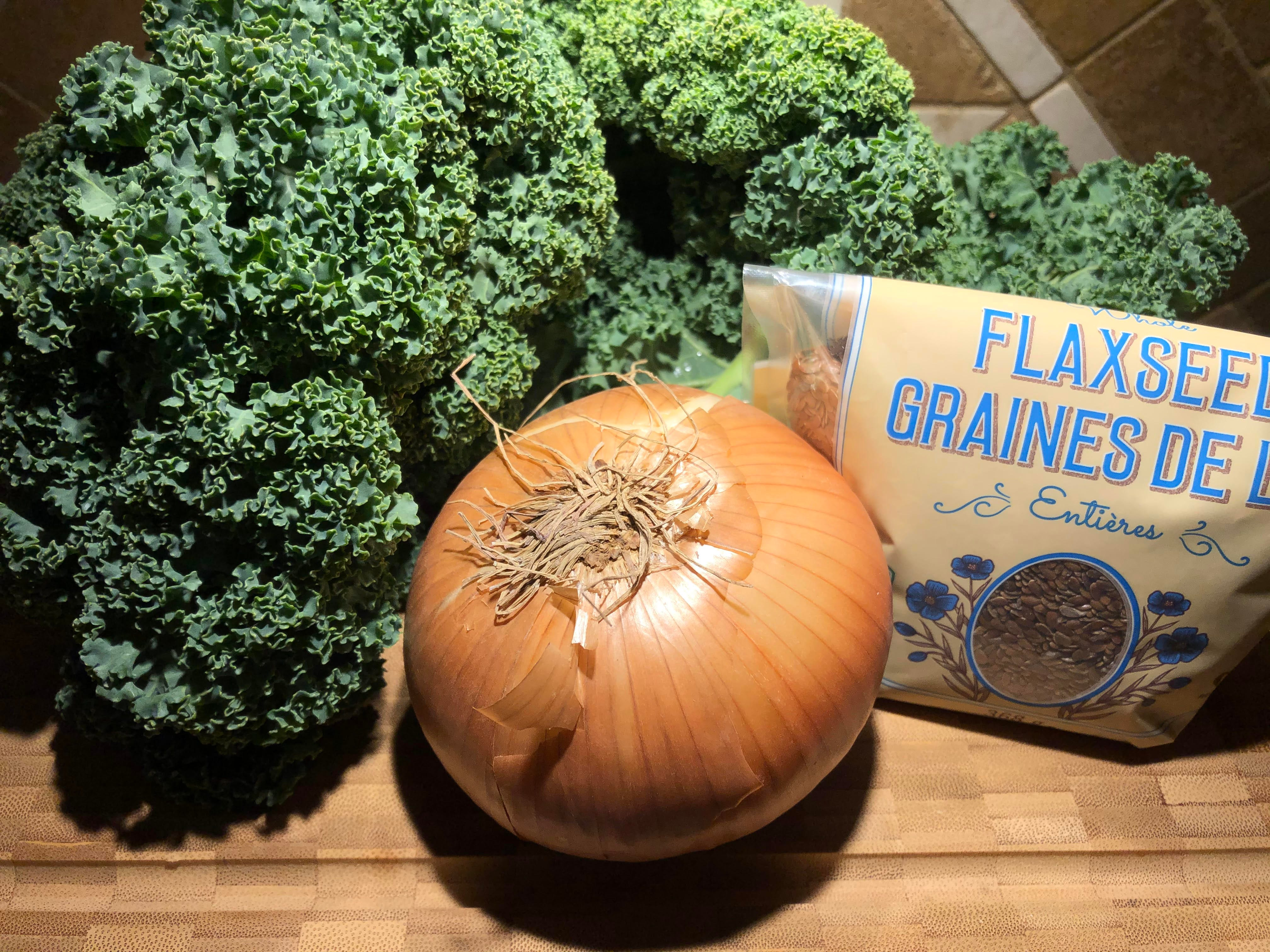Kale, flax seed, onion. Why? Read on…

# Aggregating Multiple Columns

In this article, I share a technique for computing ad-hoc aggregations that can involve multiple columns. This technique is easy to use and adapt for your needs, and results in code that’s straight forward to interpret.

So what do I mean by “multiple column agg”?

Consider some function `y(col1; col2, col3, …)` where col1 is a discreet field to group by and the additional columns are metrics involved in the aggregation.

One such example would be the Pearson correlation coefficient. In this example, my function `y` above is called as `r`, with `col1 -> x` and `col2 -> y`.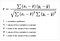Pearson correlation coefficient

Later on I’ll show how this can be calculated with pandas.

# Pandas Groupby example using '`apply`'

There is extensive documentation on how groupby can be used. If you’re working in Jupyter then go ahead and try `pd.DataFrame.groupby().<tab>` to see a huge list of functions and attributes with which to perform calculations.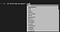Groupby functions & attributes

The idea of this blog post is to show you how to use the apply function.

Starting with some example data…

`import pandas as pdimport numpy as npdf = pd.DataFrame(np.random.random((100, 3)), columns=list('xyz'))df["a"] = np.random.choice(["kale", "flax seed", "onion"], size=100)`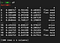Sample DataFrame

Now let’s do some arbitrary groupby calculations to demonstrate the featured technique in this post:

`df.groupby("a").apply(lambda s: pd.Series({     "x sum": s["x"].sum(),     "y mean": s["y"].mean(),     "(x+z) mean": (s["x"]+s["z"]).mean(), }))`

Pretty neat eh?

Just for fun, let’s implement the correlation coefficient (as seen above)

`p_corr = lambda x, y: (     ((x-x.mean()) * (y-y.mean())).sum()     / np.sqrt((((x-x.mean())**2).sum() * ((y-y.mean())**2).sum())) )df.groupby("a").apply(lambda s: pd.Series({    "corr(x, y)": p_corr(s["x"], s["y"]),    "corr(x, z)": p_corr(s["x"], s["z"]),}))`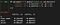Pearson correlation coefficients

Nothing stopping you from using numpy’s implementation instead (it’s probably much faster, after all). Go ahead and check my work ;) This should result in a pivot table with the same values as that above:

`df.groupby("a").apply(lambda s: pd.Series({    "corr(x, y)": np.corrcoef(s["x"], s["y"]),    "corr(x, z)": np.corrcoef(s["x"], s["z"]),}))`

# Conclusion

I found this little trick the other week when I was working on a web-data analysis for a client. I fell in love immediately — and I hope that you have as well.

But I’m wondering.. does this bring to mind any tricks that you know? What is your favorite way to use groupby for data analysis. Let me know in a comment or on twitter.

As always, thanks for reading. Now get back to your work! Your projects are missing you ;)

https://alexgalea.ca/

Python Data Engineer, MSc. Physics

## More from Alex Galea

Python Data Engineer, MSc. Physics